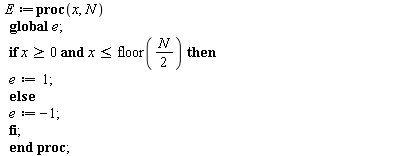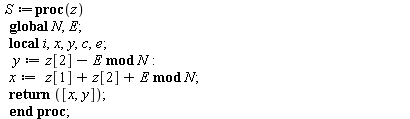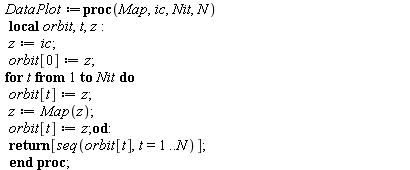## 30 Reputation

6 years, 166 days

## How to plot in maple?...

Maple

Hello,

I am trying to plot this map

y:=y[t+1] +e(x[t]) mod N
x:=x[t]+y[t+1] mod N

where e(x[t]) is a piecewise function defined by

e(x[t])=1 if 0<=x< floor(N/2) OR

e(x[t])=-1 if floor(N/2) <=x<N
for any initial conditions [x,y] and N (but must be integers).
What I did is two separate functions: 1) for e(x[t]) functiion is given by

E := proc (x, N) global e; if 0 <= x and x <= floor((1/2)*N) then e := 1 else e := -1 end if end procand 2) for the main function

S := proc (z) local i, x, y, c, e; global N, E; y := `mod`(z-E, N); x := `mod`(z+z+E, N); return [x, y] end procMy orbit procedure (so that the output is list of integers [x,y]) is given by:This DataPlot does not give the list output of integers. I do not know whether this function is correctly written.
Can somebody help me producing the list of the orbit [x,y]?
Thank you very much for helping!

## Chirikov standard map...

Maple

Hi everyone,

I am trying to plot the standard map equations which is defined by

p_{n+1}=p_{n}+k sin x_{n}  (mod 2pi)
x_{n+1}=x_{n}+p_{n}+k sin x_{n} (mod 2pi).

Is there is any simple code for plotting this map in Maple?

## How to categorize the output...

Maple

Hello,

I need help to classify my output. If somebody can help me to do that. I want to write the procedure MipEq where
I am interested to extract the first entries for every lists and categorize them. If the second entries are the same, then I want to classify the first entries into one output. Say, I have this output:

>MipEq(10);
{[0,[1,4,2,1]],[1,[2,1,4,2]],[2,[3,6,4,3]],[3,[3,6,4,3]],[4,[4,2,1,4]],[5,[4,3,6,4]],[6,[4,3,6,4]],[7,[5,8,6,5]],[8,[5,8,6,5]],[9,[5,8,6,5]],[10,[6,4,3,6]]}.

The bold output, its second entries are the same. Therefore I want to classify all the first entries so that it doesnt repeat.

I would like to have the new output:

{[0,[1,4,2,1]],[1,[2,1,4,2]],[[2,3],[3,6,4,3]],[4,[4,2,1,4]],[[5,6],[4,3,6,4]],[[7,8,9],[5,8,6,5]],[[10,[6,4,3,6]]}.

If the output is quite complicated to obtain, I can provide the procedures that involved in MipEq.
There are:
for parameter a,b:=3,1;

The function F:

> Fp := proc (x) local Up, up; global a, b; Up := simplify((1/2)*(2*b-a+sqrt((2*b-a)^2+8*a*x))/a); if type(Up, integer) then up := Up+1 else up := ceil(Up) end if; return x+2*up*b-a*up^2 end proc;
> Fm := proc (x) local Um, um; global a, b; Um := simplify((1/2)*(-2*b-a+sqrt((2*b+a)^2-8*a*x))/a); um := ceil(Um); return x+2*um*b+a*um^2 end proc;
> F := proc (x) global Fp, Fm; Fm(Fp(x)); return % end proc;

The orbitPeriodic:

>OrbitPeriodic := proc (Map, ic) local orbit, t, z; z := ic; orbit := z; for t while z <> ic or t = 1 do orbit[t] := z; z := Map(z); orbit[t] := z end do; return [seq(orbit[t], t = 0 .. t-1)] end proc;

The code:

>C := proc (x, Intvl) local i, n; n := nops(Intvl); if x = 0 then return 1 else for i from 2 to n do if x < Intvl[i] and Intvl[i-1] < x then return i-1 end if; if x = Intvl[i] then return i end if end do end if; "ERROR! YOU HAVE TO ADD THE INTERVALS" end proc;

Intervals:

> X := proc (m::integer) local D; D := (1/2)*a*m^2-b*m+(1/2)*a*m; return % end proc;
> Xp := proc (m::integer) local d; if 2*b < a then d := (1/2)*a*m^2-3*b*m+(3/2)*a*m-2*b+a else d := (1/2)*a*m^2+(7/2)*a*m-3*b*m+4*a-4*b end if; return % end proc;
>E := {seq(X(m), m = 0 .. 200)}; H := {seq(Xp(m), m = 0 .. 200)}; Intvl := `union`(E, H);

Equivalence Classes:

MipEq := proc (N) local Used, MinPts, IcCode, AllCodes, Codes, AC, q, ic, x, z, RT, AllPoints, Extract, EqClasses, EqClasses1; global F, C, OrbitPeriodic; Used := array(0 .. N); MinPts := array(0 .. N); IcCode := array(0 .. N); for ic from 0 to N do MinPts[ic] := true; for x in OrbitPeriodic(F, ic) do if x <= N then Used[x] := true end if end do end do; for ic from 0 to N do OrbitPeriodic(F, ic); IcCode[ic] := [ic, map(C, %, Intvl)] end do; Codes := {seq(IcCode[ic], ic = 0 .. N)}; AC := {seq(IcCode[ic], ic = 0 .. N)}; AllCodes := convert(AC, 'list'); RT := select(proc (x) options operator, arrow; `in`(x, AllCodes) end proc, Codes); return Codes, AllCodes end proc;

I hope somebody can help me.
Thank you very much for the help!

## Loop with boolean ...

Maple

Hi everyone,

Can anybody help me to write a programme in maple? I want to do a loop that iterate from an integer from 0 to N where I want to write a prodedure, say, MEC(N). Then I want to organise the output into a list of three lists  [[min points],[escape],[capture]], which, if correct, should include all integers from 0 to N exactly once.

> MEC := proc (N)
local IsEmpty, Escape, escape, capture, minpoints, Capture, z, MinPts, ic;
IsEmpty := array(0 .. 2*N);
Escape := array(0 .. 2*N);
Capture := array(0 .. 2*N);
MinPts := array(0 .. N);
IsEmpty := true;
Escape := true;
Capture := true;
MinPts := true;

for ic from 0 to N do
if IsEmpty then
z := ic;
MinPts[ic] := true;
z := ic;
while z <= 2*N and F(z) <= 2*N do
z := F(z);
IsEmpty[z] := true;
Escape[z] := true
end do;

z := ic;
while z <= 2*N and Finv(z) <= 2*N do
z := Finv(z);
IsEmpty[z] := true;
Capture[z] := true end do fi;
end do;
return [MinPts(z), Escape(z), Capture(z)]
end proc;

There are two operations involved in the program which is the function F and F inverse called Finv.
i need to produce the output which contain the list of integers exactly once.

I could not figure out which logical expression that I do wrong.

## Maple procedure for the first return ma...

Maple

I want to do the procedure for the FirstReturn of a map. I think my procedure is not correct.
I want to write a procedure
FirstReturn:=proc(F,x,p,q) where F is any map (function), x is the integer and the starting point, and p&q is the integer that form an interval [p,q].
If i start from the point x in [p,q], then after some iterations, there is Ft(x) in the interval [p,q].
I am interested with the the value of Ft(x) and the iteration, t when it comes back to the interval [p,q]  to be the output of the procedure.
What i did is shown below. But I dont have any idea to make it work!

FirstReturn := proc (F, x, p, q)
local t, z;
if p <= x and x <= q then F(x) := x end if;
for t while x < p and q < x or t = 1 do
x := z;
if p <= z and z <= q then
z;
end if;
end do;
return (z, t);
end proc;

 1 2Page 1 of 2
﻿